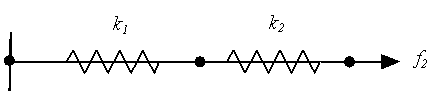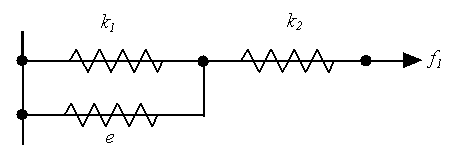# # Ill Conditioning

In the vast majority of cases the solver will give a correct solution to the problem. However, some problems are by nature ill-conditioned in which case small changes in the input data can lead to more significant changes in the results.

Taking a simple example to look at ill-conditioning; consider a simple two spring system, where the springs are connected in series. The stiffness of the first spring is and that of the second is and we assume that is much greater than .In this case the equations describing the system is

As in a solver based on a Gaussian elimination technique, we use these equations to arrive at a relationship between and

which when substituted in the other equation gives:

or

With exact arithmetic the term would be zero, however, if is large compared with and due to limited precision, some error will be introduced in the calculation. If this error is denoted by , then the equation we have is

We have then a system as shown below where the error is like adding a third spring, which acts in parallel with .The expected reaction is , but the reaction that is calculated is

Thus the reaction is in error by a factor